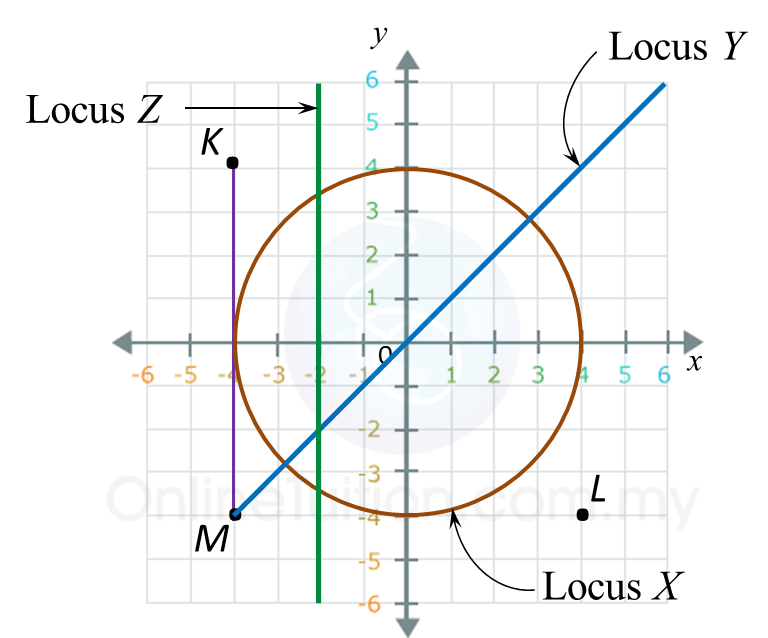# 8.2.4 Loci in Two Dimensions, PT3 Focus Practice

 
                 
Question 7:
 
Diagram in the answer space below shows a polygon. X and Y are two moving points in the polygon.
 
 (a) On the diagram, draw
 (i) the locus of the point X such that XQ = XR.
 (ii) the locus of the point Y such that YQ = QR.
 
 (b) Hence, mark with the symbol                                 the intersection of the locus of X and the locus of Y.   
 
 
 Answer:                   
(a)(i), (ii) and (b)Solution:
 (a)(i), (ii) and (b)Question 8:
 
Diagram below shows a Cartesian plane.
 Draw the locus of X, Y and Z.
 
 (a) X is a point which moves such that its distance is constantly 4 units from the origin.
 
 (b) Y is a point which moves such that it is always equidistant from point K and point L.
 
 (c) Z is a point which moves such that it is always equidistant from lines KM and y-axis.
 
 Answer:Solution: